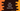# Dart remove items from a list that doesn't satisfy a condition## Dart example to remove items from a list that doesn’t satisfy a condition:

This post will show you how to remove all items from a list in dart. For example, if the given list is [1, 2, 3, 4, 5], and if we remove all even numbers from this list, it will become [1, 3, 5].

It can be done simply by iterating through the values of the list one by one and removing the items which are not required.

But, there is one more method defined in dart list that is used only for removing all elements from a list that doesn’t satisfy a given condition. It is retainWhere. In this post, we will learn how to use retainWhere with example.

### Definition of retainWhere:

retainWhere is defined as below:

``void retainWhere(bool test(E e))``

This method removes all the elements from a list that fail to satisfy the function test.

### Error:

It will throw one error if the list is a fixed length list. It throws UnsupportedError.

### Example 1: Remove all odd numbers from a dart list:

Let’s take an example of retainWhere:

``````void main() {
var items = [1, 2, 3, 4, 5, 6, 7, 8, 9, 10];
items.retainWhere((e) => e % 2 == 0);

print(items);
}``````

For this example, we are removing all elements which are not divisible by 2, i.e. odd numbers.

If you run the above program, it will print the below output:

``[2, 4, 6, 8, 10]``

### Example 2: Remove items from a list of objects:

We can also use retainWhere to remove items from a list of objects. For example, let’s take a look at the below program:

``````void main() {
var items = [
{"name": "Alex", "age": 20},
{"name": "Bob", "age": 21},
{"name": "Chandler", "age": 16}
];
items.retainWhere((e) => (e["age"] as double) % 2 == 0);

print(items);
}``````
• It has one list of custom objects called items
• Using retainWhere, we are removing all items with age value not equal to 0.

It will print the below output:

``[{name: Alex, age: 20}, {name: Chandler, age: 16}]``